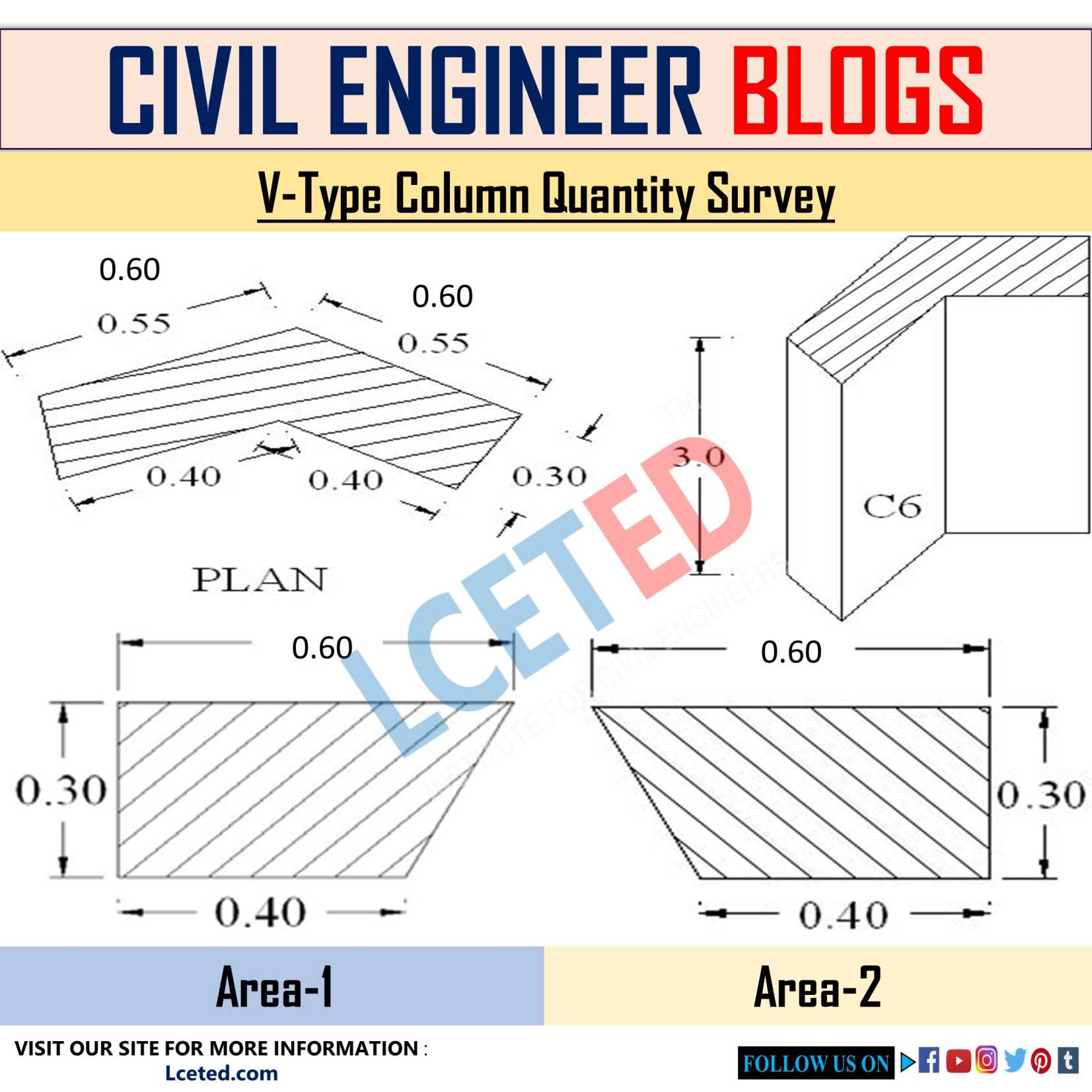V-Type Column Quantity Survey | Volume Of Concrete | Area Of Shuttering | Area Of Paint -lceted LCETED INSTITUTE FOR CIVIL ENGINEERS

## Nov 11, 2021

V-Type Column Quantity Survey | Volume Of Concrete | Area Of Shuttering | Area Of Paint

1. V‐ type Column Volume Of Concrete Calculation

2. V‐type Column Area Of Shuttering Calculation

3. V‐type Column Area Of Paint CalculationV-Type Column Quantity Survey

Solution:

1. Volume of Concrete = Area x Depth

To find the area of column divide area in to two parts

L1 = 0.60;

L2 = 0.40 and

B = 0.30

Average L =( L1 + L2)/2

L = (0.60 + 0.40) /2

L = 0.5m

Therefore,

Area1 = L x B = 0.5 x 0.30 = 0.15

Area2 = L x B = 0.5 x 0.30 = 0.15

Total area = 0.30 m2

Volume of Concrete = Area x Depth

= 0.30 x 3.0

= 0.9 m3

2. Shuttering Area = Peripheral length x Depth

Therefore Peripheral length = 0.60 + 0.60 + 0.30 + 0.40 + 0.40 +0.30 = 2.60m

= 2.60 x 3.0

= 7.8 m2

3. Area of paint = Peripheral Length x Depth

= 2.60 x 3.0

= 7.8 m2

If you find